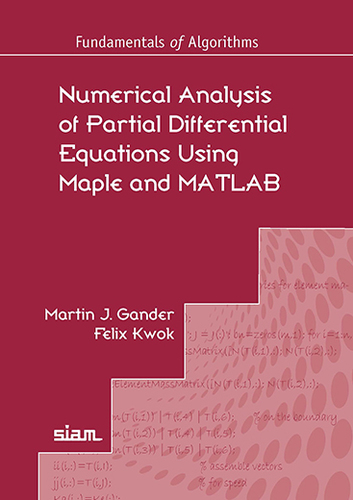Numerical Analysis of Partial Differential Equations Using Maple and MATLAB Martin J. Gander, Felix Kwok Published by SIAM
Home Summary Content Programs Authors

 Select: Home Summary Content Programs Authors
 :: Table of Contents Chapter 1. Introduction    1.1 Notation    1.2 Ordinary Differential Equations (ODEs)    1.3 Partial Differential Equations (PDEs)    1.4 The Heat Equation    1.5 The Advection Reaction Diffusion Equation    1.6 The Wave Equation    1.7 Maxwell's Equations    1.8 Navier-Stokes Equations    1.9 Elliptic Problems    1.10 Problems Chapter 2. The Finite Difference Method    2.1 Finite Differences for the Two Dimensional Poisson Equation    2.2 Convergence Analysis    2.3 More Accurate Approximations    2.4 More General Boundary Conditions    2.5 More General Differential Operators    2.6 More General, Non-Rectangular Domains    2.7 Room Temperature Simulation Using Finite Differences    2.8 Concluding Remarks    2.9 Problems Chapter 3. The Finite Volume Method    3.1 Finite Volumes for a General Two Dimensional Diffusion Equation    3.2 Boundary Conditions    3.3 Relation Between Finite Volumes and Finite Differences    3.4 Finite Volume Methods are not Consistent    3.5 Convergence Analysis    3.6 Concluding Remarks    3.7 Problems Chapter 4. The Spectral Method    4.1 Spectral Method Based on Fourier Series    4.2 Spectral Method with Discrete Fourier Series    4.3 Convergence Analysis    4.4 Spectral Method based on Chebyshev Polynomials    4.5 Concluding Remarks    4.6 Problems Chapter 5. The Finite Element Method    5.1 Strong Form, Weak or Variational Form, and Minimization    5.2 Discretization    5.3 More General Boundary Conditions    5.4 Sobolev Spaces    5.5 Convergence analysis    5.6 Generalization to Two Dimensions    5.7 Where are the Finite Elements ?    5.8 Concluding Remarks    5.9 Problems

Home Summary Content Programs Authors